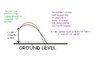# Angle when a projectile is launched at a height from the ground

This a question from projectile motion. This is NOT "homework"; it's a concept that got me thinking.

When a projectile is launched from the ground level and it falls back on the same (ground) level, the horizontal displacement is maximised if the angle of projection (with respect to the horizontal) is 45 degrees.

BUT when the projectile is launched from a height above the ground, and it falls onto the ground level, the maximum horizontal displacement is covered if the angle of projection is slightly less than 45 degrees.

My question is, what relation can we derive to determine the angle theta (w.r.t. the horizontal) at which the projectile should be launched from that height h so as to get maximum horizontal displacement M? That is, what is the relation between theta, h, R and M as per the illustration (attached)?
R is the horizontal displacement if the angle of projection at h were 45 degrees.

#### Attachments

•projectile_motion.JPG
22.5 KB · Views: 497

The basic equations you need to work with are (ignoring air resistance):
0 = h + vtsinθ - gt2/2 (process stops when you hit the ground)
d = vtcosθ

d = horizontal distance, t = time of flight, v = initial speed of projectile, g = accceleration due to gravity

Eliminate t to get d as a function of θ. Use calculus to maximize d.

physwizard
Try this:
##sin \alpha = \frac{1}{\sqrt{2 - \frac{2gh}{u^2}}}##

(Negative value of 'h' needs to be applied for points below. )

(I know I am not supposed to post answers here but I guess it is easier than telling you how to work it out.)

Last edited:
Staff Emeritus
Gold Member
This a question from projectile motion. This is NOT "homework"; it's a concept that got me thinking.

But it certainly could be, so to the homework forum with it.